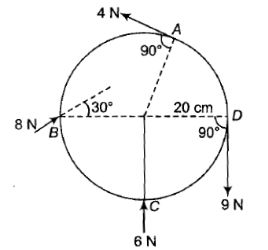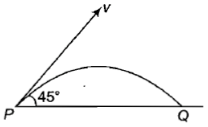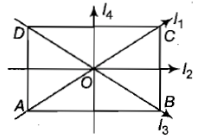A particle of mass 15 kg has an initial velocity m/s. It collides with another body and the impact time is 0.1 s, resulting in a velocity m/s after impact. The average force of impact on the particle is

1.

2.

3.

4.

Concept Questions :-

Linear momentum
High Yielding Test Series + Question Bank - NEET 2020

Difficulty Level:

Two Circular discs A and B are of equal masses and thicknesses but made of metal with densities (). If their moments of inertia about an axis passing through their centers and perpendicular to circular faces be , then

1.

2.

3.  ${\mathrm{I}}_{\mathrm{A}} < {\mathrm{I}}_{\mathrm{B}}$

4.

Concept Questions :-

Moment of inertia
High Yielding Test Series + Question Bank - NEET 2020

Difficulty Level:

A body is rolling without slipping on a horizontal surface and its rotational kinetic energy is equal to the translational kinetic energy. The body is

1.  Disc

2.  Sphere

3.  Cylinder

4.  Ring

Concept Questions :-

Moment of inertia
High Yielding Test Series + Question Bank - NEET 2020

Difficulty Level:

A wheel of radius 20 cm forces applied to it as shown in the figure. The torque produced by the forces 4 N at A, 8 N at B, 6 N at C at D at angles indicated is1.  5.4 N-m anticlockwise

2.  1.80 N-m clockwise

3.  2.0 M-m clockwise

4.  3.6 n-m clockwise

Concept Questions :-

Torque
High Yielding Test Series + Question Bank - NEET 2020

Difficulty Level:

A projectile of mass m is fired with velocity v from a point P, as shown. Neglecting air resistance, the magnitude of the change in momentum between the points P and arriving at Q is1.  Zero

2.  $\frac{\mathrm{mv}}{\sqrt{2}}$

3.

4.  2 mv

Concept Questions :-

Linear momentum
High Yielding Test Series + Question Bank - NEET 2020

Difficulty Level:

The moment of inertia of a thin rectangular plate ABCD of uniform thickness about an axis passing through the centre O and perpendicular to the plane of the plate is1.

2.

3.

4.

Concept Questions :-

Moment of inertia
High Yielding Test Series + Question Bank - NEET 2020

Difficulty Level:

The centre of a wheel rolling on a plane surface moves with a speed ${\mathrm{v}}_{0}$. A particle on the rim of the wheel at the same level as the centre will be moving at speed

1.  zero

2.  ${\mathrm{v}}_{0}$

3.  $\sqrt{2}{\mathrm{v}}_{0}$

4.  $2{\mathrm{v}}_{0}$

Concept Questions :-

Rolling motion
High Yielding Test Series + Question Bank - NEET 2020

Difficulty Level:

A man weighing 80 kg is standing in a trolley weighing 320 kg. The trolley is resting on frictionless horizontal rails. If the man starts walking on the trolley with a speed of 1 m/s, then after 4 sec his displacement relative to the ground will be

(1) 5  m

(2) 4.8 m

(3) 3.2 m

(4) 3.0 m

Concept Questions :-

Center of mass
High Yielding Test Series + Question Bank - NEET 2020

Difficulty Level:

Moment of inertia of a uniform cylinder of mass M, radius R and length l about an axis passing through its center and normal to its axis would be

1.  $\frac{{\mathrm{Ml}}^{2}}{12}$

2.  $\frac{{\mathrm{MR}}^{2}}{2}$

3.

4.

Concept Questions :-

Moment of inertia
High Yielding Test Series + Question Bank - NEET 2020

Difficulty Level:

A particle moves along a circle of radius $\frac{20}{\mathrm{\pi }}m$ with constant tangential acceleration. If the velocity of the particle is 80 m/s at the end of the second revolution after motion has begin, the tangential acceleration is

(1) 640

(2) 160

(3) 40

(4) 40 $\mathrm{m}/{\mathrm{s}}^{2}$

Concept Questions :-

Rotation motion : Introduction